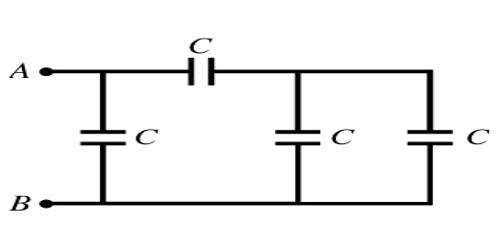# Equivalent Capacitance

Equivalent Capacitance

Capacitance is the ability of a system to store an electric charge, and it is measured in farads. If a capacitor is used in lieu of a combination of capacitors so that the charge in a plate and the potential difference between the plates of the capacitor is equal to the charge and potential difference of the combination of capacitors, then the capacitance of that capacitor is called the equivalent capacitance.A capacitor is a device in a circuit which stores charge. So, a capacitor with a large capacitance will store more charge than one with a smaller capacitance. You charge a capacitor by connecting it to a battery, and you discharge it by removing the battery and connecting it to another circuit component.